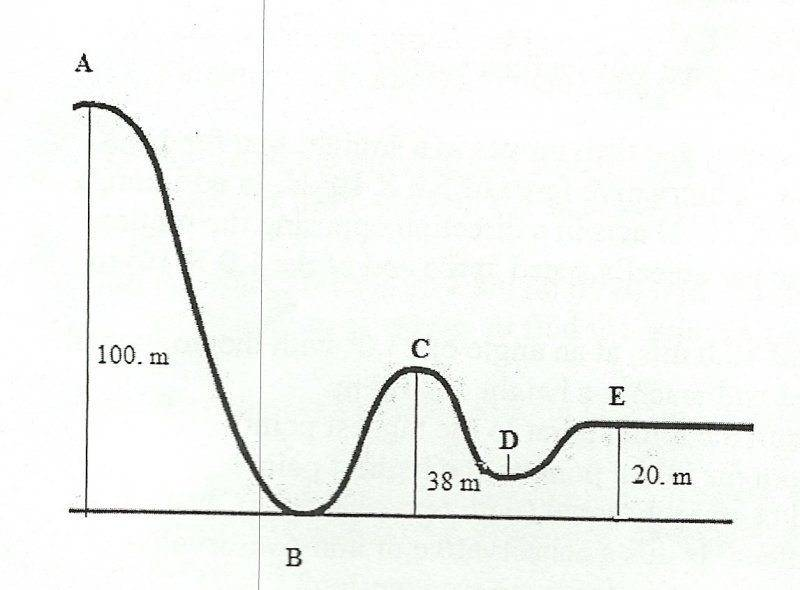Why do we ignore normal forces when applying conservation of energy to roller coasters?

Consider this setup to study conservation of energy:It is usually taught that the potential energy at any point on this roller coaster is given by:

$$U =mgh$$

Where $h$ is the height above the ground.

But the gravity is not the only force here. There is friction due to the the surface of the roller coaster, then there is air resistance, and finally the normal force to the surface of the roller coaster (the one that keeps it from falling down).

We can neglect friction and air resistance to make the problem simpler but how can we neglect the normal force? I am clearly missing something, can someone help?

• I don't understand how taking into account any kind of force, be it normal force or friction, can affect the equation for the potential energy. – Dmitry Grigoryev Jan 22 '18 at 15:08
• Never mind, I got the answer. Check the accepted answer. – Anonymous Jan 22 '18 at 15:22
• The normal force is always perpendicular to the direction of motion (by definition) therefore it does no work and doesn't take away energy. – immibis Jan 22 '18 at 20:46

There are two things you need to take into account

Work/Energy

The 'conservation of energy' is just a consequence of the work energy theorem, which basically states that net work on a particle equals the change in its kinetic energy

$$W = \Delta K = \frac{1}{2}mv_B^2 - \frac{1}{2}mv_A^2$$

Where the quantity $W$ is calculated as

$$W = \int_{A}^B{\rm d}{\bf x}\cdot{\bf F}$$

The important thing you should notice here is the operation '$\cdot$' (dot product): If the displacement is perpendicular to the force there's not work done! That means that the normal will not contribute to $W$

Friction

Of course friction will contribute, you just need to calculate the above integral for it, but usually is neglected in this type of problems

• Got it, the dot product is key. – Anonymous Jan 22 '18 at 10:12
• We mainly neglect friction because its nasty to estimate and the integral tends to become elliptical once you include it and drag. Which can not be solved without a computer. – joojaa Jan 22 '18 at 20:23

The power done by a force is given by:

$$P = \vec F\cdot \vec v = \frac{dW}{dt}$$

Since the normal force is always perpendicular to the velocity, $P=0$, and the normal force does no work. All it does is change the direction of motion- it never changes the speed in this sort of problem. (It does, of course, change the speed when an object hits the ground, but there $\vec v$ and the normal force are not perpendicular).

• Your disclaimer makes me think of Rincewind: "It's not the fall I'm afraid of, it's the ground at the end" – Tobias Kienzler Jan 22 '18 at 10:56

The kinetic energy theorem relates the difference in kinetic energy $K$ to the work $W$ exerted by all forces

$$\Delta K = W$$

In the case of the roller coaster, gravity is working (and due to gravity being a conservative force we know $W=-mg\Delta h$. The normal force, however, is (as the name states) always normal to the motion of the object so its work is $0$ (remember that work of a force, in a simplified version, $F$ is $W=Frcos(\theta)$ where $r$ is the space travelled by the object and $\theta$ is the angle between the force and the motion, in the case of the normal force $\theta=90°$ so the work is vanishing.

If there is friction and/or air resistance, they might have a component of force in the direction of motion leading to work against the motion.

It is interesting to note that if you observe the same system in your picture form a moving reference frame, you would see the rollercoaster (hence the object) moving too in the direction of your motion and in that case the normal force will not be perpendicular to the motion of the object and thus will exert work - thus energy can be different in different reference frames. But that's another thing..!

The work done by a force is $W=\int \vec{F}\cdot d\vec{s}$, and the fact that this is a scalar product is important, because it means that the angle matters. The normal force is perpendicular to the movement, so it does not do work.

Since $\Delta E_k= \Delta W$, or $\Delta E = \Delta W_{NC}$; it won't change the total energy because it does no work.

However, the normal force affects friction by $F_{fr}=\mu\cdot N$. This time friction acts in the direction of movement and it can do work (in fact it always take energy away from the body), but we usually neglect this fact, as much as we do with air friction.